Symbol
ProblemSolve the following problems. $EXpla1n$ how you arrived at your answers. $1.$ A rectangular garden has an area of $84m^{2}and$ a perimeter of $38m$ Find $nd$ its length and width. $2.$ A children's park is $350mlon$ long and $200m$ wide. It is surrounded $loy$ a pathway of uniform width. Suppose the total area of the park and the $pathway$ is $74,464m^{2}.$ $HoM$ wide is the pathway? $3.$ A $car$ travels $20$ kph faster than a truck. The car $COVerS$ $350km$ in $twO$ hours less than the time it takes the truck to travel the same distance. What is the speed of the car? How about the truck?
7th-9th grade
Algebra
Search count: 105
SolutionQanda teacher - Ashritha
1.) Let s = the speed of the truck and (s+20) = the speed of the car to get the time of each vehicle time = distance/ speed truck time = 350/s car time = 350/(s+20) Truck time - car time = 2 hrs so, (350/s) - [350/(s+20)] = 2 LCD we can get [350(s+20) - 350s]/s(s+20) = 2 then transpose the denominator 350(s+20) - 350s = 2s(s+20) simplifying  we can get 350s + 7000 - 350s = 2s^2 + 40s then simplify more to become quadratic equation 2s^2 + 40s - 7000 = 0 to make it simplest divide the equation by 2 s^2 + 20s - 3500 = 0 by factoring we can get s= 50 s= -70 (impossible because of the sign) so s = 50 km/h is the speed of the truck s + 20 = 70 km/h is the speed of car
3rd answer is above text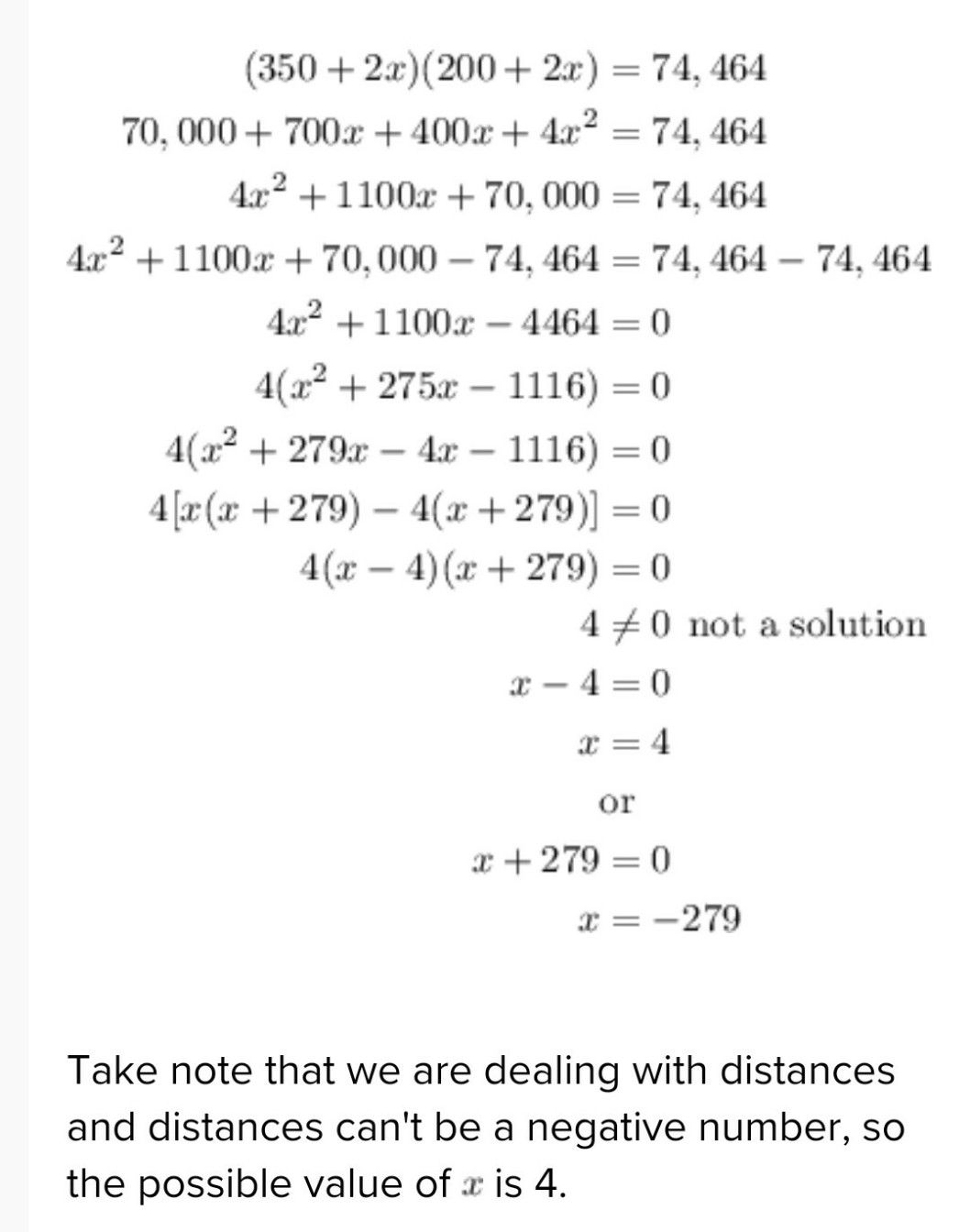2nd answer in above picture
first we'll get the answer using arithmetic and then using quadratic equations 38/2=19, half of the perimeter 84=2*2*3*7=(2*2*3)*(7)=12*7 and 12+7=19 length and width are 7 and 12 or 12 and 7 (meters) P=2l+2w 38=2l+2w 19=l+w l=19-w A=lw 84=(19-w)w 84=19w-w2 w2-19w+84=0 factor now, you factor 84 which is what I did at the top... 84=(2*2*3)*(7) (w-12)(w-7)=0 w-12=0 w=12 (and l=7) or,... w-7=0 w=7 (and l=12)
3rd answer in above text
I didn't have notebook with me present so i sent you text answer if you want a written answer you can ask me i will send you later if you want
sorry last text is 1st answerStudent
thank you so much maam.Qanda teacher - Ashritha
you are welcomeStudent
can i know your name on facebook? because I need someone to teach me this is my last coins and I do not know how to get coins
by the way im grade 9 studentQanda teacher - Ashritha
actually i am really sorry we are not allowed to share our personal information so i can't help you with this
please understandStudent
oh its fine i just need someone to teach meQanda teacher - Ashritha
okay thanks for understandingStudent
can you be my tutor only here?Qanda teacher - Ashritha
yes but i can't answer for the questions here because it in our rules we can't answer here for more questions you have to ask seperatelyStudent
its ok thank you! ♥️Qanda teacher - Ashritha
?☺️
please evaluate the answerStudent
hi maam
can i ask something?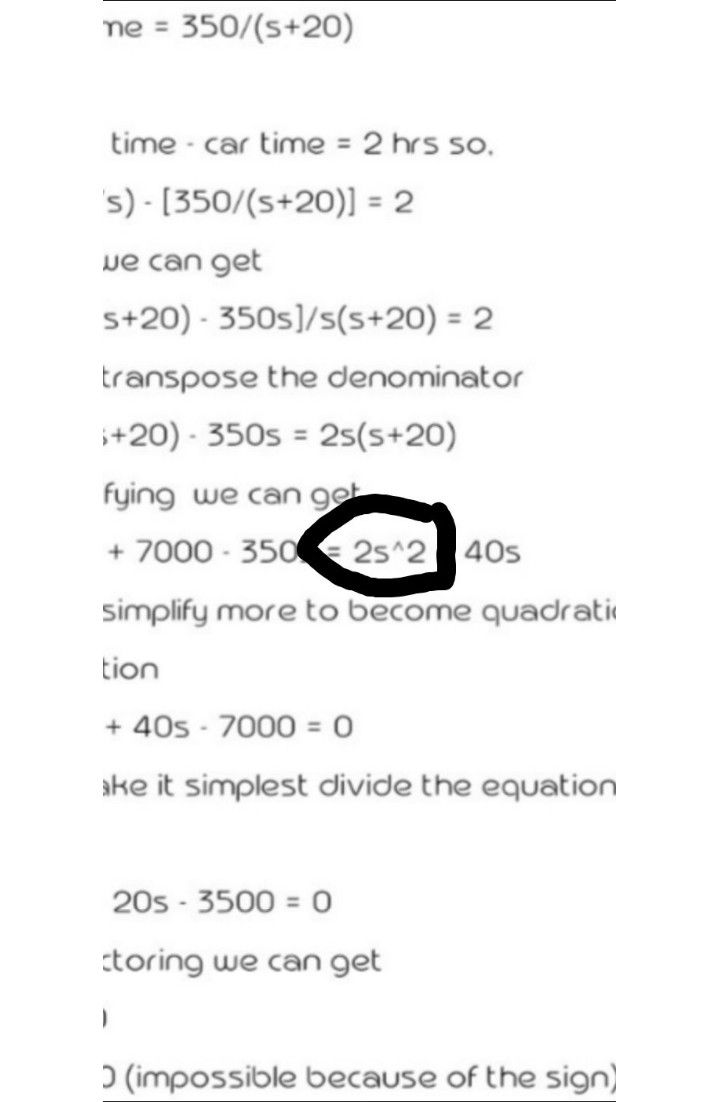is the number I circled like this?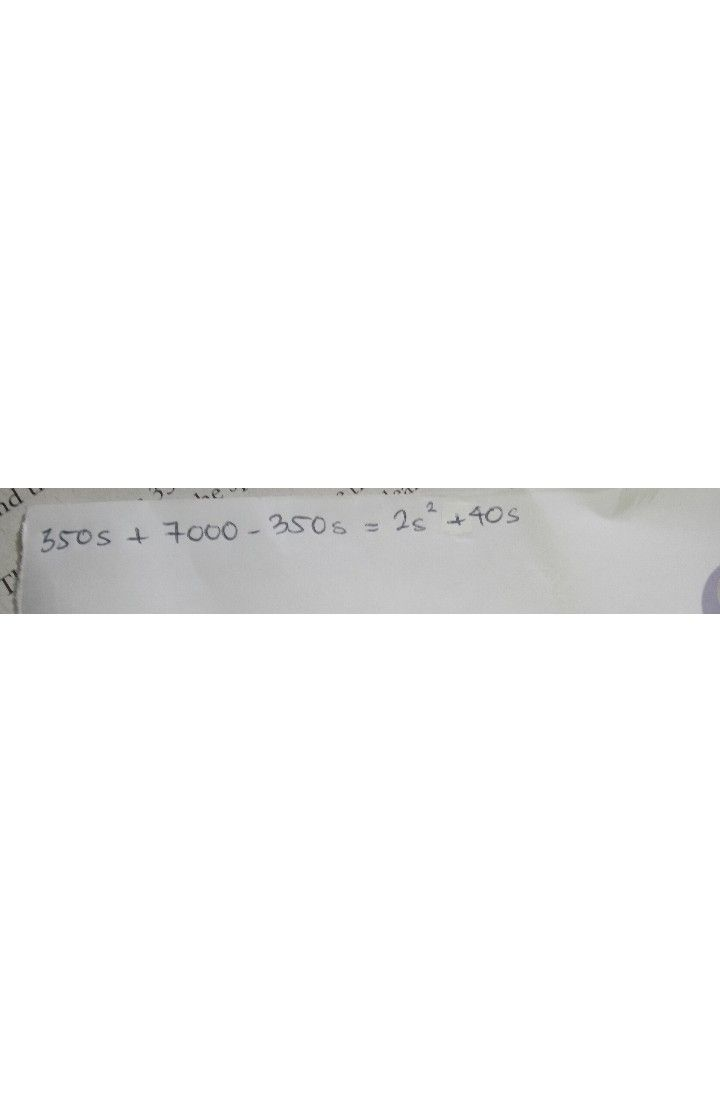i just need your answer right nowQanda teacher - Ashritha
i didn't understand?
what?Student
you send me this answer
1.) Let s = the speed of the truck and (s+20) = the speed of the car to get the time of each vehicle time = distance/ speed truck time = 350/s car time = 350/(s+20) Truck time - car time = 2 hrs so, (350/s) - [350/(s+20)] = 2 LCD we can get [350(s+20) - 350s]/s(s+20) = 2 then transpose the denominator 350(s+20) - 350s = 2s(s+20) simplifying  we can get 350s + 7000 - 350s = 2s^2 + 40s then simplify more to become quadratic equation 2s^2 + 40s - 7000 = 0 to make it simplest divide the equation by 2 s^2 + 20s - 3500 = 0 by factoring we can get s= 50 s= -70 (impossible because of the sign) so s = 50 km/h is the speed of the truck s + 20 = 70 km/h is the speed of carQanda teacher - Ashritha
yes what is your doubt in thatStudent
is the s^2 is like this? s²Qanda teacher - Ashritha
yes its s squareStudent
ok thank youQanda teacher - Ashritha
you are welcomeStudent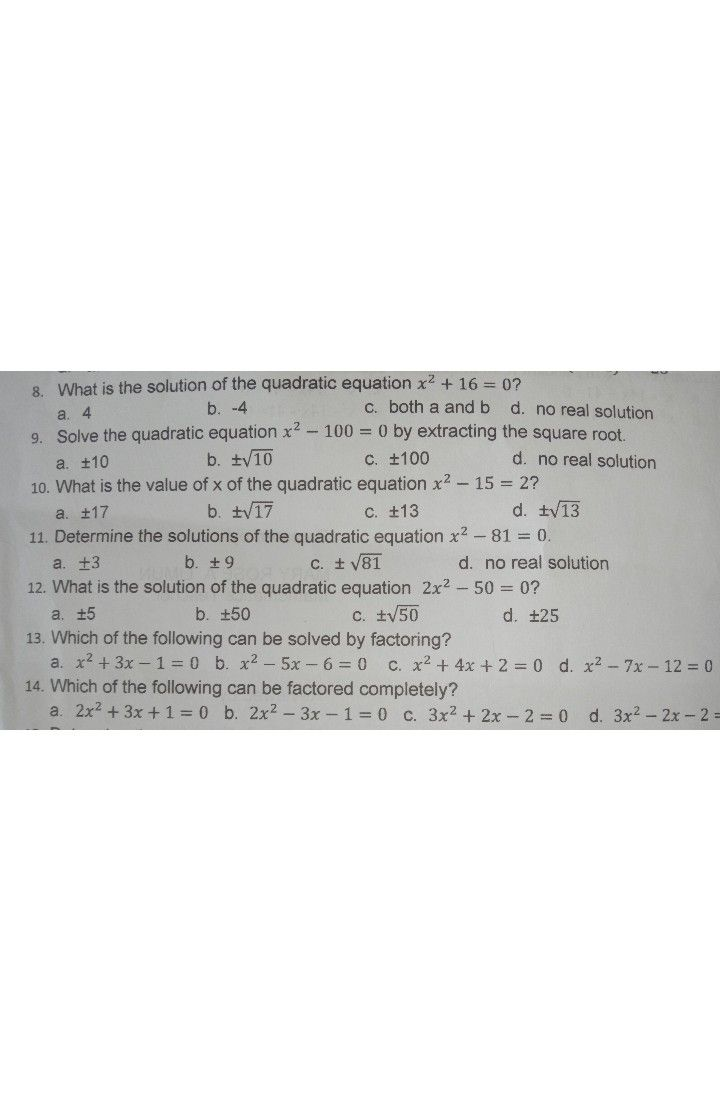encircle the correct answer i ask the 7 questions in the other teacher
maam please help me with thisQanda teacher - Ashritha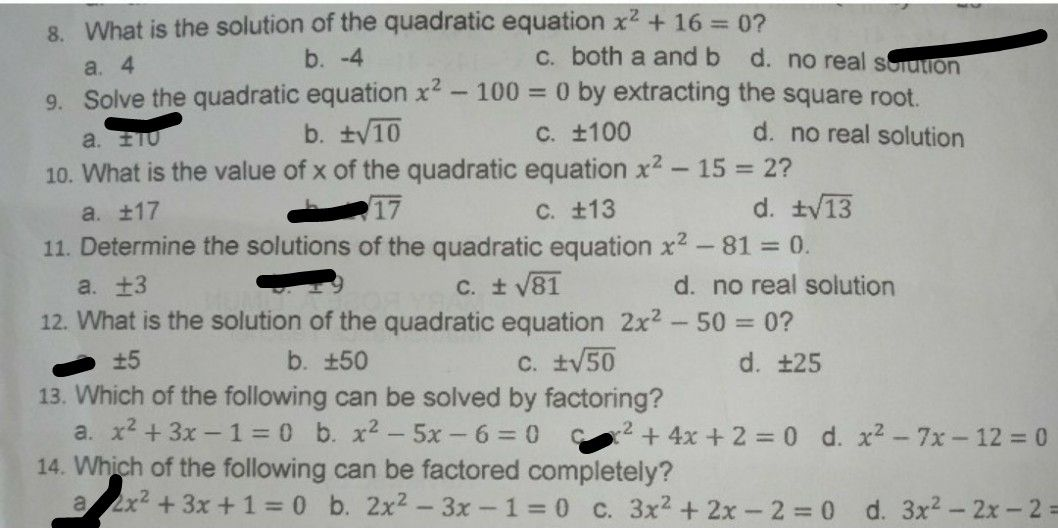Student
thank you so much maam! ❤️Qanda teacher - Ashritha
you are welcome
please evaluate the answer